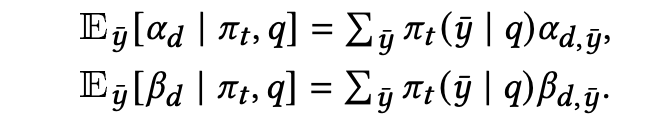# Computing expectation with information across different tensors

Background: I am working with a Plackett-Luce model in a learning-to-rank setting, which means for a given query q, I have N sampled rankings, where each ranking has a d number of documents. Assuming there is max ‘d’ documents to be ranked.

For each ranking in a given query, I have a probability of the given ranking, i.e. a tensor ranking_prob of dimension [#query, #ranking_samples].

From the model, for each query, I have sampled N rankings, where each ranking is of size d, i.e. I have a tensor sampled_ranking of dimensions: [#query, #ranking_samples, #documents].I want to basically compute this expectation. For each document d, in a ranking y, the alpha value is based on the rank of the document in the ranking y. I have a fixed alpha vector, where each index is the ranking position.

Now with these tensors, I don’t know how to compute this expectation. The information is spread across different tensors, and I don’t know how to aggregate them.